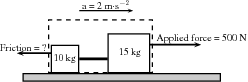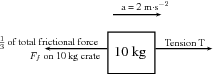# 11.2 Newton's second law  (Page 2/3)

 Page 2 / 3

In a similar investigation where the mass is kept constant, but the applied force is varied, you will find that the bigger the force is, the faster the object will move. The acceleration of the trolley is therefore directly proportional to the resultant force. In mathematical terms:

$a\propto F$

Rearranging the above equations, we get $a$ $\propto$ $\frac{F}{m}$ OR $F\phantom{\rule{3.33333pt}{0ex}}=\phantom{\rule{3.33333pt}{0ex}}ma$

Newton formulated his second law as follows:

Newton's Second Law of Motion

If a resultant force acts on a body, it will cause the body to accelerate in the direction of the resultant force. The acceleration of the body will be directly proportional to the resultant force and inversely proportional to the mass of the body. The mathematical representation is:

$F=ma.$

## Applying newton's second law

Newton's Second Law can be applied to a variety of situations. We will look at the main types of examples that you need to study.

A 10 kg box is placed on a table. A horizontal force of 32 N is applied to the box. A frictional force of 7 N is present between the surface and the box.

1. Draw a force diagram indicating all the horizontal forces acting on the box.
2. Calculate the acceleration of the box.
1. We only look at the forces acting in a horizontal direction (left-right) and not vertical (up-down) forces. The applied force and the force of friction will be included. The force of gravity, which is a vertical force, will not be included.

2. We have been given:

Applied force F ${}_{1}$ = 32 N

Frictional force F ${}_{f}$ = - 7 N

Mass m = 10 kg

To calculate the acceleration of the box we will be using the equation ${F}_{R}=ma$ . Therefore:

$\begin{array}{ccc}\hfill {F}_{R}& =& ma\hfill \\ \hfill {F}_{1}+{F}_{f}& =& \left(10\right)\left(a\right)\hfill \\ \hfill 32-7& =& 10\phantom{\rule{3.33333pt}{0ex}}a\hfill \\ \hfill 25& =& 10\phantom{\rule{3.33333pt}{0ex}}a\hfill \\ \hfill a& =& 2,5\phantom{\rule{0.166667em}{0ex}}\mathrm{m}·{\mathrm{s}}^{-1}\phantom{\rule{3pt}{0ex}}\mathrm{towards the left}\hfill \end{array}$

Two crates, 10 kg and 15 kg respectively, are connected with a thick rope according to the diagram. A force of 500 N is applied. The boxes move with an acceleration of 2 m $·$ s ${}^{-2}$ . One third of the total frictional force is acting on the 10 kg block and two thirds on the 15 kg block. Calculate:

1. the magnitude and direction of the frictional force present.
2. the magnitude of the tension in the rope at T.
1. Always draw a force diagram although the question might not ask for it. The acceleration of the whole system is given, therefore a force diagram of the whole system will be drawn. Because the two crates are seen as a unit, the force diagram will look like this:Force diagram for two crates on a surface
2. To find the frictional force we will apply Newton's Second Law. We are given the mass (10 + 15 kg) and the acceleration (2 m $·$ s ${}^{-2}$ ). Choose the direction of motion to be the positive direction (to the right is positive).

$\begin{array}{c}\hfill {F}_{R}=ma\\ \hfill {F}_{\mathrm{applied}}+{F}_{f}=ma\\ \hfill 500+{F}_{f}=\left(10+15\right)\left(2\right)\\ \hfill {F}_{f}=50-500\\ \hfill {F}_{f}=-450N\end{array}$

The frictional force is 450 N opposite to the direction of motion (to the left).

3. To find the tension in the rope we need to look at one of the two crates on their own. Let's choose the 10 kg crate. Firstly, we need to draw a force diagram:Force diagram of 10 kg crate

The frictional force on the 10 kg block is one third of the total, therefore:

${F}_{f}=\frac{1}{3}×$ 450

${F}_{f}=150\phantom{\rule{4pt}{0ex}}\mathrm{N}$

If we apply Newton's Second Law:

$\begin{array}{ccc}\hfill {F}_{R}& =& ma\hfill \\ \hfill T+{F}_{f}& =& \left(10\right)\left(2\right)\hfill \\ \hfill T+\left(-150\right)& =& 20\hfill \\ \hfill T& =& 170\phantom{\rule{4pt}{0ex}}\mathrm{N}\hfill \end{array}$

Note: If we had used the same principle and applied it to 15 kg crate, our calculations would have been the following:

$\begin{array}{ccc}\hfill {F}_{R}& =& ma\hfill \\ \hfill {F}_{\mathrm{applied}}+T+{F}_{f}& =& \left(15\right)\left(2\right)\hfill \\ \hfill 500+T+\left(-300\right)& =& 30\hfill \\ \hfill T& =& -170\phantom{\rule{4pt}{0ex}}\mathrm{N}\hfill \end{array}$

The negative answer here means that the force is in the direction opposite to the motion, in other words to the left, which is correct. However, the question asks for the magnitude of the force and your answer will be quoted as 170 N.

#### Questions & Answers

how to calculate net electric field at a point
what are controlled variables
Independent variables in an experiment which influence the outcome results
Boss
Such as temperature when dealing with ohms law
Boss
variables whose amount is determined by the person doing the experiment
Clemencia
how to calculate exodation number
Calculate relative atomic mass
what is chemical bonding?
Chemical Bonding is a mutual attraction between two atoms resulting from the simultaneous attraction between their nuclei and the outer elections
Sydney
How would you go about finding the resistance of an unknown resistor using only power supply, a voltmeter and a known resistance
Lebona
who is the scientist who discovered electromagnetism
what happens to the galvanometer when the switch is closed ?
Zivele
how do we identify an oxidising or reducing agent in a reaction?
Zivele
hey
Banele
what is electricity
Formula for concentration
c=m/MV
Zipho
if given number of moles and volume , can use c=n/V
sibahle
Chemistry term three topic is stressing me out, I really need a huge help
Ziyanda
on what
Olebogeng
during a snooker competition ,a 200g ball A m moving with velocity va collide head on with a identical ball B that was at rest.A after the collision ball A remains at rest wile ball B moves on with a velocity of 4m/s? With what speed was ball a moving before the collision
a vector can be resolved into a horizontal component only
how to calculate normal force
how to calculate wavelength
Lizoh
Hello. How does a real gas behave under low temperature and high pressure?
does a vector quantity include force and distance?
yes
Itumeleng
what's the difference between a vector and a scalar?
Keaobaka
vector is the physical quantity with magnitude and direction Scalar is the Physical quantity with magnitude only
Bobo
Newton's second law of motion
Newton second law motin
Shife
Newton's second law of motion: When a resultant/net force acts on an object, the object will accelerate in the direction of the force at an acceleration directly proportional to the force and inversely proportional to the mass of the object.
Bobo
newtons third law of motion
Ayakha
when object A exert a force on object B object B SIMULTANEOUS exert a force of equal magnitude on object A
Tshepiso
what is tail to tail method
it is a method used tobrepresent vectors it is a rectangle
ZiveleBy DanielrosenbergerByBy Eric CrawfordBy Mistry BhaveshByBy Anindyo Mukhopadhyay